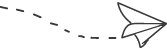# c语言实现二分法_太经典啦！14个C语言算法（附详细代码）void main(){int a,x,i,p;for(i=0;i<=11;i++)x=0;for(i=1;i<=100;i++){a=rand() % 100;printf("%4d",a);if(i%10==0)printf("\n");}for(i=1;i<=100;i++){p="a"%10;if(p==0) p="10";x[p]=x[p]+1;}for(i=1;i<=10;i++){p="i";if(i==10) p="0";printf("%d,%d\n",p,x);}printf("\n");}(1) 对于已知两数m，n，使得m>n；

(2) m除以n得余数r；

(3) 若r=0，则n为求得的最大公约数，算法结束；否则执行(4)；

(4) m←n，n←r，再重复执行(2)。

m n r

14 6 2

6 2 0

void main(){ int nm,r,n,m,t;printf("please input two numbers:\n");scanf("%d,%d",&m,&n);nm=n*m;if (m{ t="n"; n="m"; m="t"; }r=m%n;while (r!=0){ m="n"; n="r"; r="m"%n; }printf("最大公约数:%d\n",n);printf("最小公倍数:%d\n",nm/n);}void main(){ int m,i,k;printf("please input a number:\n");scanf("%d",&m);k=sqrt(m);for(i=2;iif(m%i==0) break;if(i>=k)printf("该数是素数");elseprintf("该数不是素数");}将其写成一函数,若为素数返回1，不是则返回0int prime( m%){int i,k;k=sqrt(m);for(i=2;iif(m%i==0) return 0;return 1;}验证哥德巴赫猜想

#include "math.h"int prime(int m){ int i,k;k=sqrt(m);for(i=2;iif(m%i==0) break;if(i>=k)return 1;elsereturn 0;}main(){ int x,i;printf("please input a even number(>=6):\n");scanf("%d",&x);if (x<6||x%2!=0)printf("data error!\n");elsefor(i=2;i<=x/2;i++)if (prime(i)&&prime(x-i)){printf("%d+%d\n",i,x-i);printf("验证成功!");break;}}排序问题

1．选择法排序(升序)

1)对有n个数的序列(存放在数组a(n)中)，从中选出最小的数，与第1个数交换位置；

2)除第1 个数外，其余n-1个数中选最小的数，与第2个数交换位置；

3)依次类推，选择了n-1次后，这个数列已按升序排列。

void main(){ int i,j,imin,s,a;printf("\n input 10 numbers:\n");for(i=0;i<10;i++)scanf("%d",&a);for(i=0;i<9;i++){ imin="i";for(j=i+1;j<10;j++)if(a[imin]>a[j]) imin="j";if(i!=imin){s=a; a=a[imin]; a[imin]=s; }printf("%d\n",a);}}

2．冒泡法排序(升序)

1)有n个数(存放在数组a(n)中)，第一趟将每相邻两个数比较，小的调到前头，经n-1次两两相邻比较后，最大的数已“沉底”，放在最后一个位置，小数上升“浮起”；

2)第二趟对余下的n-1个数(最大的数已“沉底”)按上法比较，经n-2次两两相邻比较后得次大的数；

3)依次类推，n个数共进行n-1趟比较，在第j趟中要进行n-j次两两比较。

void main(){ int a;int i,j,t;printf("input 10 numbers\n");for(i=0;i<10;i++)scanf("%d",&a);printf("\n");for(j=0;j<=8;j++)for(i=0;i<9-j;i++)if(a>a[i+1]){t=a;a=a[i+1];a[i+1]=t;}printf("the sorted numbers:\n");for(i=0;i<10;i++)printf("%d\n",a);}

3．合并法排序(将两个有序数组A、B合并成另一个有序的数组C，升序)

1)先在A、B数组中各取第一个元素进行比较，将小的元素放入C数组；

2)取小的元素所在数组的下一个元素与另一数组中上次比较后较大的元素比较，重复上述比较过程，直到某个数组被先排完；

3)将另一个数组剩余元素抄入C数组，合并排序完成。

void main(){ int a,b,c,i,ia,ib,ic;printf("please input the first array:\n");for(i=0;i<10;i++)scanf("%d",&a);for(i=0;i<10;i++)scanf("%d",&b);printf("\n");ia=0;ib=0;ic=0;while(ia<10&&ib<10){ if(a[ia]{ c[ic]=a[ia];ia++;}else{ c[ic]=b[ib];ib++;}ic++;}while(ia<=9){ c[ic]=a[ia];ia++;ic++;}while(ib<=9){ c[ic]=b[ib];b++;ic++;}for(i=0;i<20;i++)printf("%d\n",c);}① 顺序查找法(在一列数中查找某数x)

void main(){ int a,p,x,i;printf("please input the array:\n");for(i=0;i<10;i++)scanf("%d",&a);printf("please input the number you want find:\n");scanf("%d",&x);printf("\n");p=0;while(x!=a[p]&&p<10)p++;if(p>=10)printf("the number is not found!\n");elseprintf("the number is found the no%d!\n",p);}

② 基本思想：一列数放在数组a---a[n]中，待查找的关键值为key，把key与a数组中的元素从头到尾一一进行比较查找，若相同，查找成功，若找不到，则查找失败。(查找子过程如下。index：存放找到元素的下标。)

void main(){ int a,index,x,i;printf("please input the array:\n");for(i=0;i<10;i++)scanf("%d",&a);printf("please input the number you want find:\n");scanf("%d",&x);printf("\n");index=-1;for(i=0;i<10;i++)if(x==a){ index="i"; break;}if(index==-1)printf("the number is not found!\n");elseprintf("the number is found the no%d!\n",index);}二分法

int Dichotomy(int *ucData, int long, int num){int iDataLow  = 0 ;int iDataHigh = num - 1;int iDataMIDDLE;while (iDataLow <= iDataHigh){iDataMIDDLE = (iDataHigh + iDataLow)/2;i f (ucData[iDataMIDDLE] > long){iDataHigh = iDataMIDDLE - 1 ;}else if (ucData[iDataMIDDLE] < long){iDataLow = iDataMIDDLE + 1 ;}  else{return iDataMIDDLE ;}}}限幅滤波法

#define A 10char value;char filter(){   char new_value;new_value = get_ad();if ( ( new_value - value > A ) || ( value - new_value > A ))  return value;return new_value;}中位值滤波法

#define N   11char filter(){  char value_buf[N]， count,i,j,temp;for ( count=0;count{  value_buf[count] = get_ad();    delay();   }for (j=0;j{  for (i=0;i{  if ( value_buf>value_buf[i+1] ){temp = value_buf; value_buf = value_buf[i+1]; value_buf[i+1] = temp;  }}}return value_buf[(N-1)/2];}#define N 12char filter(){int   sum = 0，count;for ( count=0;count{  sum+=get_ad();    delay();}return (char)(sum/N);}#define N 12char value_buf[N]，i=0;char filter(){ char count; int   sum=0;value_buf[i++] = get_ad();if ( i == N )    i = 0;for ( count=0;countsum = value_buf[count];return (char)(sum/N);}#define a 50char value;char filter(){ char   new_value;new_value = get_ad();return (100-a)*value + a*new_value;}PID控制算法

PID调节规律是连续系统动态品质校正的一种有效方法，它的参数整定方式简便，结构改变灵活(PI、PD、…)。

PID调节器各校正环节的作用：

PID调节器是一种线性调节器，它将给定值r(t)与实际输出值c(t)的偏差的比例(P)、积分(I)、微分(D)通过线性组合构成控制量，对控制对象进行控制。

#include#includetypedef struct PID {double SetPoint;     // 设定目标Desired valuedouble Proportion;    // 比例常数Proportional Constdouble Integral;      // 积分常数Integral Constdouble Derivative;    // 微分常数Derivative Constdouble LastError;    // Error[-1]double PrevError;    // Error[-2]double SumError;   // Sums of Errors} PID;

double sensor (void){return 100.0; }void actuator(double rDelta){}void main(void){PID sPID;double rOut;double rIn;PIDInit ( &sPID );sPID.Proportion = 0.5；sPID.Derivative = 0.0;sPID.SetPoint = 100.0;for (;;) {rIn = sensor ();rOut = PIDCalc ( &sPID,rIn );actuator ( rOut );}}1.原理

B[x],b[x]都是二进制序列,取值0或1。

M = B[m-1]*pow(2,m-1) + B[m-2]*pow(2,m-2) + ... + B*pow(2,1) + B*pow(2,0)

N = b[n-1]*pow(2,n-1) + b[n-2]*pow(2,n-2) + ... + b*pow(2,1) + n*pow(2,0)

pow(N,2) = M

(1) N的最高位b[n-1]可以根据M的最高位B[m-1]直接求得。

n-1=k, n=k+1=(m+1)/2

n-1=k-1,n=k=m/2

(2) N的次高位b[n-2]可以采用试探法来确定。

(3) 同理，可以从高位到低位逐位求出M的平方根N的各位。

3. 实现代码

/****************************************//*Function: 开根号处理                  *//*入口参数：被开方数，长整型            *//*出口参数：开方结果，整型              *//****************************************/unsigned int sqrt_16(unsigned long M){unsigned int N, i;unsigned long tmp, ttp;   // 结果、循环计数if (M == 0)               // 被开方数，开方结果也为0return 0;N = 0;tmp = (M >> 30);          // 获取最高位：B[m-1]M <<= 2;if (tmp > 1)              // 最高位为1{N ++;                 // 结果当前位为1，否则为默认的0tmp -= N;}for (i=15; i>0; i--)      // 求剩余的15位{N <<= 1;              // 左移一位tmp <<= 2;tmp += (M >> 30);     // 假设ttp = N;ttp = (ttp<<1)+1;M <<= 2;if (tmp >= ttp)       // 假设成立{tmp -= ttp;N ++;}}return N;}12-09252
08-20
12-06
11-20124
10-21111
03-062884
05-18199
01-09
09-05
04-27
07-192534
02-14880
07-131488
05-2162
05-1815
08-2141
02-068859点击重新获取扫码支付余额充值